分布式计算_分布式计算 边缘计算 - CSDN

• 分布式计算 ——原理、算法与系统 Distributed Computing —— Principles, Algorithms, and System 不定期更新   第一章 引言 第二章 分布式计算模型   第一章 引言 分布式系统：处理器、存储器、...
分布式计算 ——原理、算法与系统
Distributed Computing —— Principles, Algorithms, and System

不定期更新
中文书翻译很烂。。。各种下标错误等。。

本读书笔记首发于：https://github.com/rsy56640/Distributed_System_Learning/tree/master/Distributed Computing Principles%2C Algorithms%2C and Systems - Kshemkalyani

第一章 引言
第二章 分布式计算模型
第三章 逻辑时间
第四章 记录全局状态与快照算法

第一章 引言
分布式系统：处理器、存储器、通信网络
1.4 与并行多处理器/多计算机系统的关系
并行系统：通过将计算任务在多个处理器之间进行分配，从而获得更高的吞吐率

多处理器系统

互联网络：Omega网络、蝴蝶网络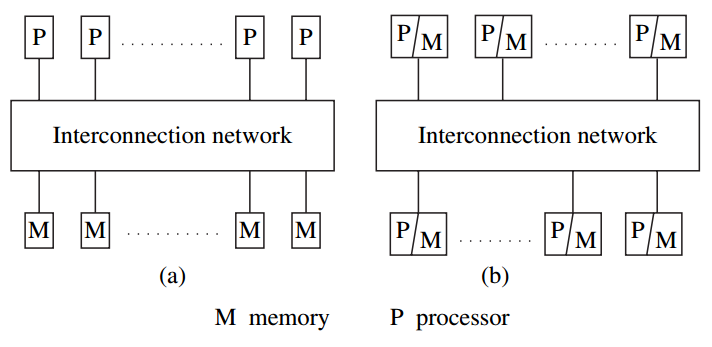图左是UMA（均匀存储器访问体系结构），右NUMA

多计算机并行系统

处理器无法直接访问共享内存
互联网络：环，超立方体

阵列处理器

基于指令流和数据流的分类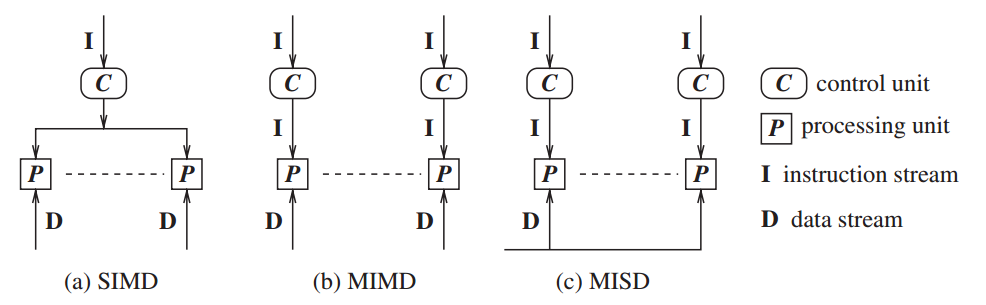1.5 消息传递系统与共享内存系统的对比

通过消息传递进行通信
通过共享内存通信

在消息传递系统上仿真共享内存
每一个共享的位置可以建模为一个隔离的进程。
处理器间通过共享内存进行通信；计算机间使用消息传递进行通信。
1.6 分布式通信的原语
消息发送原语Send()， 消息接受原语Receive()。
Send()发送方式：缓冲（拷贝到内核缓冲区，再到网络）与 非缓冲（直接拷贝到网络）。
Receive()通常采用缓冲方式。

同步原语：如果Send()和Receive()两端都实现了握手，则原语是同步的

只有调用者知道对应的Receive()原语被调用并且接受操作完成，Send()原语才算完成
当数据拷贝到接收方的用户缓冲区时，Receive()原语被认为完成

异步原语：

如果需要发送的数据被拷贝出用户缓冲区后，控制流程返回到调用进程，Send()原语被称为异步的
异步的Receive()原语无意义

阻塞原语：如果一个原语的处理完成之后控制流程返回到调用进程，则一个原语被称为阻塞的
非阻塞原语：如果一个控制流程在调用原语之后立刻返回到调用进程，甚至这个操作尚未完成，则这个原语被称为非阻塞的

怎样理解阻塞非阻塞与同步异步的区别？- 知乎
处理器同步性
同步屏障：保证在所有处理器完成前面所分配的指令之前都不会去执行下一步的代码。
1.7 同步与异步执行
异步执行

没有处理器的同步
消息延迟（传输+传播时间）是有限的
对于一个进程执行某一步任务没有时间上的上界限制

同步执行

处理器之间同步
消息分发（传输+分发时间）能够在一个逻辑步或者轮次内完成
进程执行一步具有一个已知的上界

同步系统实际上是一个特殊的异步系统——所有的通信都在其发起的轮次内完成。
1.7.3 仿真
在无错误的系统中，异步/同步 共享内存/消息传递 这4类程序可以互相仿真，即等价。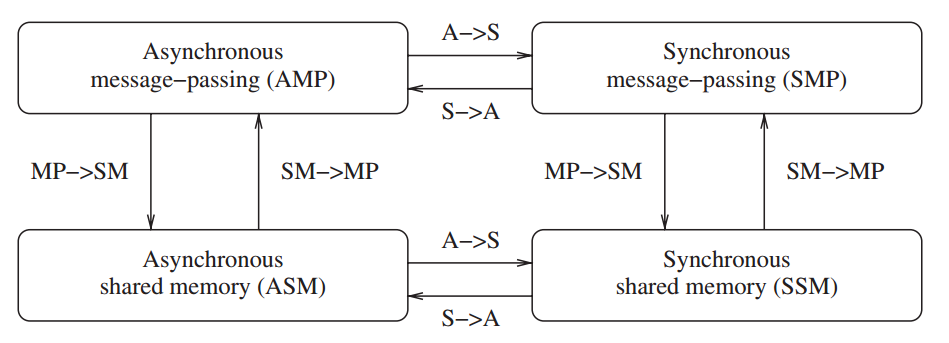但是在有错误的系统中，情况并不是这样；一个同步系统相比于异步系统具有更高的可计算性。
1.8 设计主题与挑战
1.8.1 系统角度

通信：远程过程调用、远程对象调用、面向消息的通信、面向流的通信
进程
命名：对于资源和进程的标识以及定位
数据存储与访问
一致性与复本
容错

1.8.2 算法角度

第二章 分布式计算模型
在分布式系统中，通信消息可能在传递过程中乱序、丢失、受到篡改或者重复传递。
分布式系统可以以一个有向图的方式建模，其中结点表示处理器而边则表示单向通信信道。
2.1 分布式程序
分布式程序有一组 $n$ 个异步进程 $p_1,\ p_2,\ ...,\ p_n$ 组成，令 $C_{ij}$ 表示从进程 $p_i$ 到进程 $p_j$ 的通信信道，$m_{ij}$ 表示由 $p_i$ 发往 $p_j$ 的消息。

假设每个进程都各自运行在不同的处理器上
进程间没有可共享的全局存储，而只能通过消息传递来进行联系
通信延迟是有限但无法预测的
这些进程不共享一个可随时访问的全局时钟
进程运行和消息传送是异步的

2.2 分布式运行模型
一个进程的运行可以描述为三类原子操作：内部事件、消息发送事件、消息接受事件。令 $e_i^x$ 表示进程 $p_i$ 上的第 $x$ 个事件。对一个消息 $m$，令 $send(m)$ 和 $rec(m)​$ 分别表示其发送和接收的消息。一个内部事件改变其所处的进程的状态，一个发送或接受事件改变双方的状态。
进程中的事件以出现顺序进行排序：$e_i^1,\ e_i^2,\ ...,\ e_i^x,\ ...$，该序列记为 $\mathcal{H}_i$：
$\mathcal{H}_i = (h_i, \to_i)$
其中 $h_i$ 是由 $p_i$ 产生的事件集合；二元关系 $\to_i$ 则定义了这些事件间的序。
关系 $\to_{msg}$ 表示因消息交换所导致的因果依赖关系：
$send(m) \to_{msg} rec(m)$
1. 因果优先关系
令 $H = \bigcup h_i$ 表示在一次分布式计算过程中执行的时间集合。我们在集合 $H$ 上定义一个关系 $\to$，表示事件间的因果依赖关系。
KaTeX parse error: No such environment: equation at position 93: …htarrow
\begin{̲e̲q̲u̲a̲t̲i̲o̲n̲}̲
\left\{
…
关系 $\to$ 是 Lamport 的 “happerns before” 关系。如果 $e_i \to e_j$，则事件 $e_j$ 直接或间接依赖于 $e_i$，存在着一条起始于 $e_i$，终止于 $e_j$ 的路径。
$e_i \nrightarrow e_j$ 表示事件 $e_j$ 不直接或间接依赖于 $e_i$，即事件 $e_i$ 不会对 $e_j​$ 产生因果影响。
并发：如果 $e_i \nrightarrow e_j$ 且 $e_j \nrightarrow e_i$，则事件 $e_i$ 和 $e_j$ 被称为是并发的，其关系被记为 $e_i || e_j$。
2. 逻辑并发和物理并发
两个事件是逻辑并发的，当且仅当它们之间无因果影响。与此相对，物理并发的含义是不同事件在物理时间的同一时刻发生。不论一组逻辑并发的事件是否在物理时间上同时发生，也无论它们在物理时间上实际发生的顺序如何，都不会改变计算的结果。因此。虽然一组逻辑并发的事件可能不会在物理时间的同一时刻发生，但我们总是假定这些事件在物理时间的同一时刻发生。
2.3 通信网络模型
因果依赖（CO）：对任意两个消息 $m_{ij}$ 和 $m_{kj}$，假设 $send(m_{ij}) \to send(m_{kj})$，则 $rec(m_{ij}) \to rec(m_{kj})$。确保那些发往同一目标的因果依赖的消息，以符合它们之间因果依赖关系的顺序进行发送。
通信网络模型：CO $\subset$ FIFO $\subset$ 非FIFO（随机）
因果依赖模型提供了一个内在的同步机制。
2.4 分布式系统的全局状态
分布式系统的全局状态是所有 处理器状态 和 信道状态 的集合。
处理器状态：令 $LS_i^x$ 表示处理器 $p_i$ 在事件 $e_i^x$ 发生之后，以及事件 $e_i^{x+1}$ 发生之前的状态。特别地，$LS_i^0$ 表示 $p_i$ 的初始状态。
若 $y \le x$，记为 $e_i^y \le LS_i^x$，表示 $e_i^y$ 在处理器状态之内。
信道状态：记 $SC_{ij}^{x, y}​$ 表示信道 $C_{ij}​$ 的状态：
$SC_{ij}^{x, y} = \{ m_{ij}\ |\ send(m_{ij}) \le LS_i^x\ \land\ rec(m_{ij}) \nleq LS_j^y \}$
通俗地讲，信道是消息的集合。消息 $m_{ij}$，$p_i$ 在 $x$ 之内 send；$p_j$ 在 $y$ 之后 rec。即 $SC_{ij}^{x, y} = \{ m_{ij}\ |\ m_{ij}\ 从左穿过线段e_i^{x+1} e_j^y \}$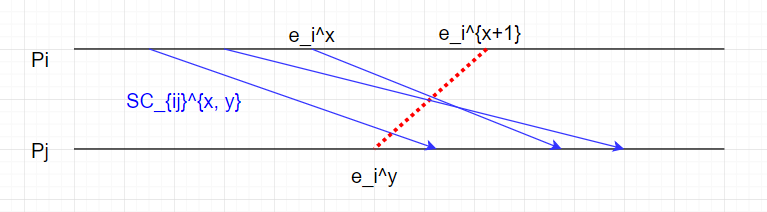全局状态：$GS = {\ \cup_iLS_i^{x_i},\ \cup_{j, k}SC_{jk}^{y_j, z_k} }$
一致性全局状态：称 $GS$ 是一致的，如果满足
$\forall m_{ij}:\ send(m_{ij}) \nleq LS_i^{x_i} \Rightarrow m_{ij} \notin SC_{ij}^{x_i, y_j}\ \land\ rec(m_{ij}) \nleq LS_j^{y_j}$

这里中文书上写错了，第一个符号写成 $\le$

通俗地讲：$p_i$ 在 $x_i$ 之后的 send，那么必须有 $p_j$ 在 $y_j​$ 之后 rec。即
$\forall m_{ij}, m_{ij}\ 不能从右穿过线段e_i^x e_j^{y+1}$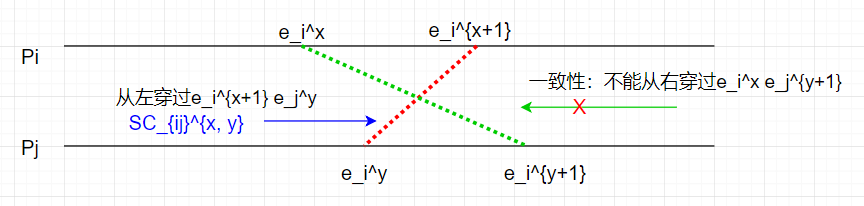非中转全局状态：所有信道为空
强一致的全局状态：一致且非中转的
2.5 分布式计算的运行分割
一条分割线 $C$ 将时空图分割为2各部分，$PAST(C)$ 表示分割线左边的所有事件的集合，$FUTURE(C)$ 表示分割线右边的所有事件的集合。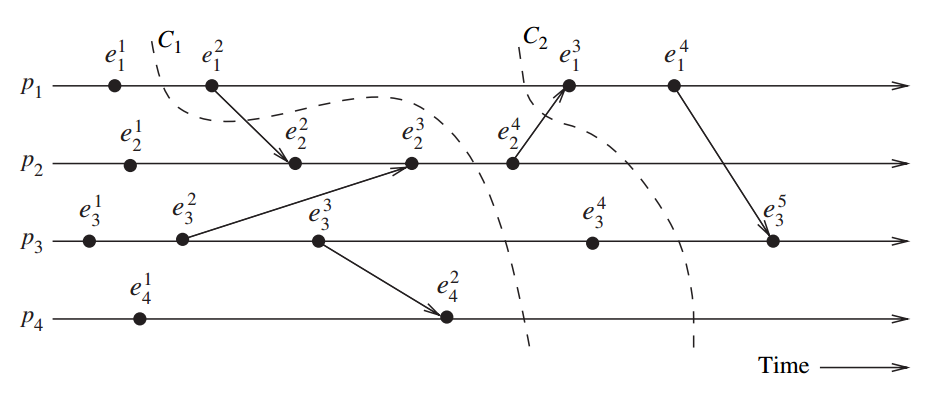$C_1$ 是非一致性分割线；$C_2$ 是一致性分割线

一致性全局状态对应一条分割线，其中每个 $PAST$ 集合中的 rec 事件都是在 $PAST$ 集中 send。
一条分割线是非一致性的，如果某个消息跨越了分割线从 $FUTURE$ 集传到 $PAST$ 集。
2.6 事件的过去和未来锥面
令 $Past(e_j)$ 表示在计算 $(H, \to)$ 中 $e_j$ 的过去事件。则，
$Past(e_j) = \{ e_i\ |\ \forall e_i \in H, e_i \to e_j \}$
记 $Past_i(e_j)$ 是进程 $p_i$ 上所有属于 $Past(e_j)$ 事件的集合。注意到 $Past_i(e_j)$ 是全序的，最大元素记为 $max(Past_i(e_j))$。注意到 $max(Past_i(e_j))$ 总是一个 send 事件。
令 $Max\_Past(e_j) = \bigcup_i \{ max(Past_i(e_j)) \}$，其包含每个进程上影响 $e_j$ 的最新事件，它被称为事件 $e_j$ 的过去锥面。
用反证法易知：$Max\_Past(e_j)$ 是一条一致性分割线。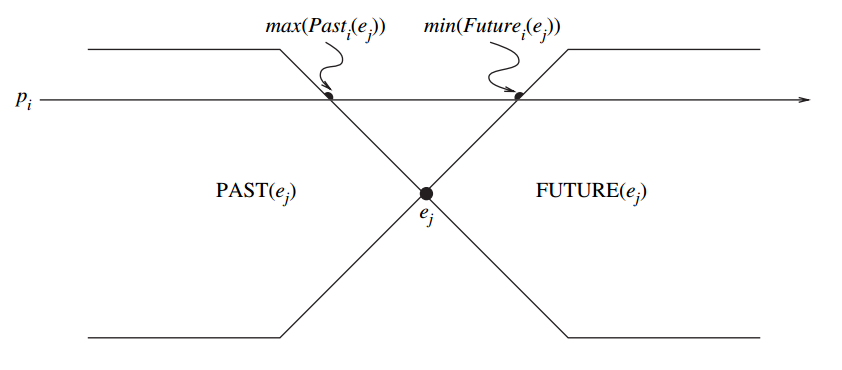类似地，事件 $e_j$ 的未来事件记作 $Future(e_j)$，它包含所有受到 $e_j$ 影响的事件 $e_i$，定义为
$Future(e_j) = \{ e_i\ |\ \forall e_i \in H, e_j \to e_i \}$
定义 $Future_i(e_j)$ 为进程 $p_i$ 上所有属于 $Future(e_j)$ 事件的集合。$min(Future_i(e_j))$ 作为 $p_i$ 上受 $e_j$ 影响的第一个事件。注意到 $min(Future_i(e_j))$ 总是 rec 事件。$Min\_Future(e_j)$ 定义为 $\bigcup_i \{ min(Future_i(e_j)) \}$，它包含了每个进程上受到事件 $e_j$ 影响的第一个事件的集合，被称为事件 $e_j$ 的未来锥面。易证它是一条一致性分割线。
在一个计算 $H$ 中，一个事件 $e$ 与 $e_j​$ 是并发的，当且仅当
$e \in H - Past(e_j) - Future(e_j)$
2.8 本章小结
进程间的消息交换显示出进程间的信息流向并且建立了进程间的因果依赖关系。进程间的优先因果关系由 Lamport 的 hanppens-before 关系确定。

第三章 逻辑时间
逻辑时间系统由一个时间域 $T$ 和一个逻辑时钟 $C$ 组成。$T$ 的元素形成关系 $<$ (happens-before) 上的偏序集合。逻辑时钟 $C$ 是一个函数，把事件 $e$ 映射到时间域 $T$ 中的一个元素，表示为 $C(e)$ 且成为 $e$ 的时间戳，定义为
$C:H\mapsto T$
使得对于事件 $e_i​$ 和 $e_j​$，有 $e_i \to e_j \Rightarrow C(e_i) < C(e_j)​$ ，这种单调性称为时钟一致性条件。
若 $e_i \to e_j \Leftrightarrow C(e_i) < C(e_j)$，则这个系统称为强一致的。
每个进程 $p_i$ 维护数据结构：

本地逻辑时钟：$lc_i$，表示进程 $p_i$ 的进度
全局时钟：$gc_i$，表示进程 $p_i$ 从本地视角所见的全局逻辑时间

更新数据结构的协议：

R1 规则：管理当进程执行一个事件时，如何更新本地逻辑时钟
R2 规则：管理进程如何更新全局逻辑时钟，使得全局进展和进程所见的全局时间得以更新

3.3 标量时间
3.3.1 定义
时间域 $T = N$，进程 $p_i$ 的本地逻辑时钟和本地全局时钟用同一个整数 $C_i$ 表示。
(1) R1 规则：
在执行一个事件之前，进程 $p_i$ 执行如下动作：
$C_i := C_i + d, \quad d > 0$
通常 $d$ 会有不同的值，典型的值保持为 1.
(2) R2 规则：
每个消息附加有它的发送方在发送时的时钟值，当进程 $p_i$ 接收到一个带有时间戳 $C_{msg}$ 的消息时，它执行如下动作：

$C_i := max(C_i, C_{msg});$
执行 R1 规则
传递该消息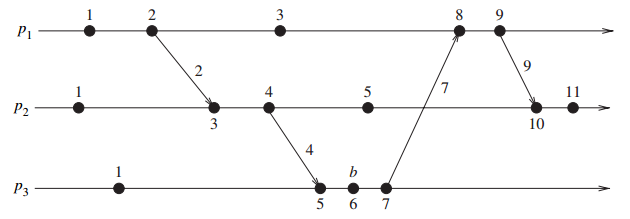3.3.2 基本性质
1. 一致性（consistency）
单调性蕴含了一致性。
2. 全序（total ordering）
注意到 $C(e_i) = C(e_j) \Rightarrow e_i || e_j$
定义时间戳为 $(t, i)$，其中 $t$ 是本地逻辑时钟，$i$ 是进程号。
全序关系 $\prec$ 定义如下：
KaTeX parse error: Expected 'EOF', got '\or' at position 36: …arrow (h < k)\ \̲o̲r̲\ ((h = k)\ \la…
注意到 $x\prec y \Rightarrow x\to y \ \lor \ x||y$，这没啥用。
3. 事件计数
如果 $d$ 总是1，则标量时间有如下性质：对于一个事件 $e$ 和时间戳 $h$，在这之前一定顺序产生了 $h-1$ 个事件，不管是哪些进程产生的。
4. 非强一致性
$C(e_i) < C(e_j) \nRightarrow e_i \to e_j$
本地逻辑时钟和本地全局时钟被压缩成一个，导致了不同进程的事件之间因果依赖关系的缺失。
3.4 向量时间
3.4.1 定义
时间域 $T = N^n$，每个进程维护一个向量 $vt_i[1..n]$，其中 $vt_i[j]$ 表示进程 $p_i$ 的有关 $p_j$ 本地时间的最近信息。用整个向量 $vt_i$ 代表 $p_i$ 所见的全局时间，并且用于给事件打上时间戳。
(1) R1 规则：
在执行一个事件之前，进程 $p_i$ 更新其本地逻辑时钟如下：
$vt_i[i] := vt_i[i] + d$
(2) R2 规则：
把每个消息 $m$ 加入到发送方进程在发送时的向量时钟 $vt$。一旦接收到这样一个消息 $(m, vt)$，进程 $p_i$ 执行如下一系列动作：

更新它的全局逻辑时间如下：

$vt_i[k] := max(vt_i[k], vt[k]),\quad k\in[1, n]$

执行 R1 规则
传送消息 m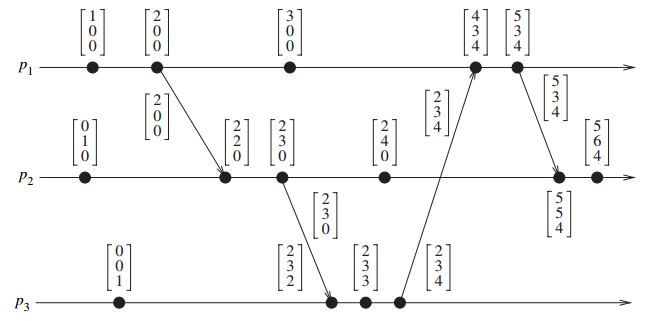3.4.2 基本性质
1. 同构
$(H, \to)$ 同构于 $(T, <)​$
\begin{aligned} e_i^x \to e_j^y & \Leftrightarrow vx < vy \\ e_i^x\ ||\ e_j^y & \Leftrightarrow vx \ ||\ vy \\ \\ e_i^x \to e_j^y & \Leftrightarrow vx[i]\le vy[i] \\ e_i^x\ ||\ e_j^y & \Leftrightarrow (vx[i] > vy[i]) \ \land\ (vx[j] < vy[j]) \\ \end{aligned}
2. 强一致性
3. 事件计数
3.5 向量时钟的有效实现
时间戳长度过大，需要优化
3.5.1 Singhal - Kshemkalyani 的差量技术
当 $p_i$ 向 $p_j$ 发送消息时，将现在的向量与上一次发送给 $p_j$ 的向量做差，为 $0$ 的忽略，其余的标记位置和当前值打包发送。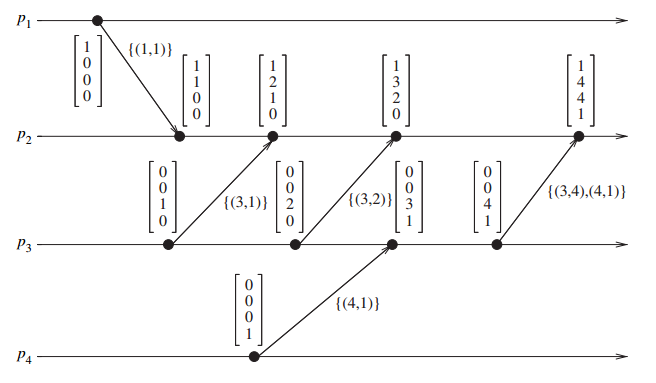即 $p_i$ 向 $p_j$ 发送 $\{(i,v)\}$，$p_j$ 更新向量如下：
$vt_j(i_k) = max(vt_i[k], v_k)$
每个进程需要记录上一次发给其他所有进程的时间戳向量。这个技术的主要价值在于将进程的存储空间开销降低到 $O(n)$，方式如下：
进程 $p_i$ 维护两个向量：

LastSent: $LS_i[1..n]$，$LS_i[j]$ 表示当 $p_i$ 上一次发送消息给 $p_j$ 时 $vt_i[i]$ 的值
LastUpdate: $LU_i[1..n]$，$LU_i[j]$ 表示当 $p_i$ 上一次更新 $vt_i[j]$ 项时 $vt_i[i]$ 的值

显然有 $LU_i[i] = vt_i[i]$，并且只有 rec 导致更新 $vt_i[j]$ 时 $LU_i[j]$ 才需要更新；只有 $p_i$ send $pj$ 时 $LS_i[j]$ 需要更新。
因此，从上次 $p_i$ 到 $p_j$ 通信以来，向量时钟中只有 $vt_i[k]$ 改变，其中 $k$ 满足 $LS_i[j] < LU_i[k]$。于是 $p_i$ 发送 $p_j$ 的集合为：
$\{\ (x, vt_i[x])\ |\ LS_i[j] < LU_i[x]\ \}$
通俗地讲：记录上次通信以来本地时钟的改变，因为 $rec$ 操作总是会更新本地时钟。
3.5.2 Fowler - Zwaenepoel 的直接依赖技术

中文版怎么回事？？？各种下标错误，我才看到P51，都有好几处错误了。还有不少翻译很迷。。

通俗地讲：只计算直接依赖，也就是通过消息同步的事件，或者本地事件。
每个进程维护一个依赖向量 $D_i[1..n] = \{0\}$，按如下方式更新：

$p_i$ 发生事件时，$D_i[i] := D_i[i] + 1$
当 $p_i$ 给 $p_j$ 发送消息时，加入更新过的 $D_i[i]$
当 $p_i$ 从 $p_j$ 接收消息时，更新 $D_i[j] := max(D_i[j], d)$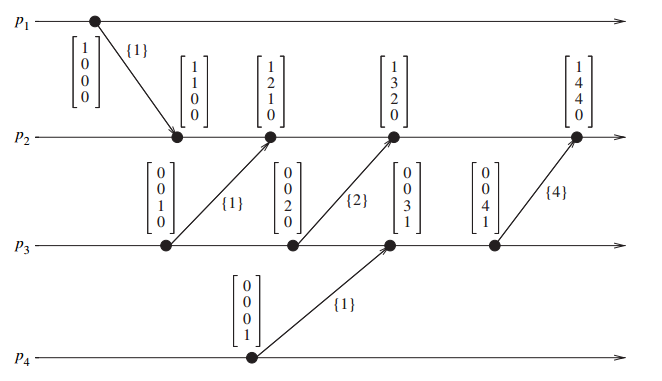依赖向量 $D_i$ 仅仅反应直接依赖。（图中 $p_2$ 直接依赖于 $p_3$，$p_3$ 直接依赖于 $p_4$，但 $p_2$ 不知道自己间接依赖于 $p_4$）
方法：间接依赖可以通过脱机的递归跟踪事件的直接依赖向量来获得。
这个方法适用于不要求频繁计算传递依赖的应用，如因果断点和异步检查点的恢复等离线计算。
离线计算算法：递归地更新过去锥面。
缺点：如果事件频繁的发生，该技术需要记录大量事件的历史。
3.6 Jard - Jourdan 的自适应技术
3.7 矩阵时间
3.7.1 定义
$T = N_{n \times n}$，进程 $p_i$ 维护一个矩阵 $mt_i[1..n][1..n]$
$mt_i[i][i]$ 表示 $p_i$ 本地逻辑时钟；
$mt_i[i][j]$ 表示 $p_i$ 具有的有关进程 $p_j$ 的本地逻辑时钟的最新知识；
$mt_i[j][k]$ 表示 $p_i$ 具有的有关进程 $p_j$ 的知识，该知识是 $p_j$ 具有的 $p_k$ 本地逻辑时钟的最新知识；
通俗地讲：就是存储了所有 $vt_i[1..n]$
(1) R1 规则：
在执行一个事件前，进程 $p_i$ 更新本地逻辑时钟：
$mt_i[i][i] := mt_i[i][i] + d$
(2) R2 规则：
每个消息附带矩阵时间 $mt$，当 $p_i$ 收到 $p_j$ 的消息 $(m,mt)$ 时，$p_i$ 执行如下：

更新矩阵如下：

\begin{aligned} mt_i[i][k] & := max(mt_i[i][k], mt[j][k]),\quad k\in [1,n] \\ mt_i[k][l] & := max(mt_i[k][l], mt[k][l]),\quad k,l\in [1,n] \end{aligned}

执行 R1 规则
发送消息 m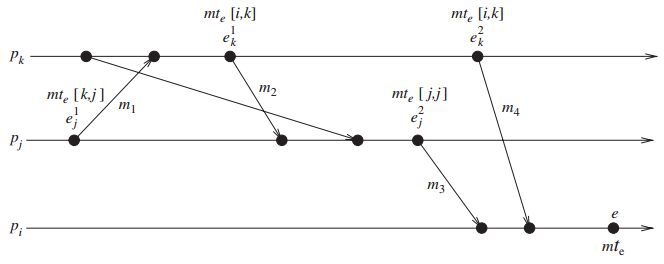通俗地讲：总是保证 $mt_i[i][.] \ge mt_i[j][.],\quad j \neq i$；即总是保证自己关于其他进程的知识在自己的矩阵时间里是最新的。
3.7.2 基本性质
$mt_i[i][.]$ 包含了向量时钟的所有性质。此外还具有如下性质：
$min_k(mt_i[k][l] \ge t) \Rightarrow\$进程 $p_i$ 知道 “每个进程知道 $p_l$ 本地时间的进展，直到 $t$”，这意味着进程 $p_i$ 知道 “所有其他进程知道 $p_l$ 不会发送本地时间 $\le t$ 的消息”，据此可以做一些优化。
3.8 虚拟时间
3.8.1 虚拟时间的定义
虚拟时间是分布式计算中一个全局的、一维的临时坐标系统，以测量计算的进展和定义同步。
消息：发送方，虚拟发送时间，接收方，虚拟接收时间。

规则 1：每个消息的虚拟发送时间 $<$ 该消息的虚拟接收时间

规则 2：进程中事件的虚拟时间是递增的

感觉像是标量时间到向量时间的一种过渡，但是表达力不及向量时间。

3.8.2 与 Lamport 逻辑时钟的比较
在虚拟时间中，时钟是按照乐观方式前进的，一旦检测到违反的情况就会采取纠正行动。
3.8.3 时间变形机制
消息的虚拟接收时间被认为是其时间戳。
时间变形机制的组成：

本地控制机制：保证按正确的次序执行事件和处理消息
全局控制机制：处理全局进展、终止检测、I/O错误处理、流控制等

3.8.4 本地控制机制
进程只有本地时钟，没有全局时钟。
一个消息的虚拟发送时间为发送方的时钟。
虚拟时间的语义要求输入消息严格按时间戳次序被每个进程接收。实现该要求的唯一方法是：在收到一个迟到的消息时，接收者回滚到一个较早的一个虚拟时间，撤销其间产生的一切附带影响，然后通过合适的顺序顺序执行这个迟到的消息并再次向前执行。
分布式系统中的回滚因这样的原因而复杂：要回滚的进程可能已经给其他进程发送了很多消息，而其他进程有可能因此产生其他事件，这就导致了多层次的附带影响。
反消息和回滚机制
进程运行时的组成如下：

进程名：唯一的虚拟空间坐标
逻辑时钟：虚拟时间坐标
进程状态
状态队列：进程状态的拷贝
输入队列：包含按虚拟接收时间顺序到达的消息。输入队列中已被处理的消息并不删除，而是加一个负号（反消息）一遍将来回滚。
输出队列：含有进程最近按虚拟发送时间顺序发送消息的反消息拷贝。在回滚时需要这些拷贝。

反消息：与消息内容相同，符号相反。传递消息时，该消息的一个拷贝进入接收方的输入队列，一个负拷贝留在发送方的输出队列以便发送方回滚。
当一个消息与其反消息出现在同一个队列中时，它们立刻无效。
回滚的触发：如果消息时间戳 $<$ 接收方本地时间，则接收方必须进行回滚。
回滚机制：

搜索状态队列，找出最大的并且 $<$ 消息时间戳的状态，并从状态队列中去除该事件之后保存的所有状态，然后从该点恢复向前执行。为了收回一条消息，只需要发送其反消息即可。
考虑反消息的接收方：

如果原消息已经到达，但还未被处理，则它的虚拟接收时间必定大于接收方的虚拟时间。反消息到来后不会引起回滚，它将直接与原消息一同无效。
如果原消息已经开始被处理，反消息的到来会使得接收方回滚到原消息被接收的虚拟时间，然后废除原消息，使接收方不保留原消息的记录。这一回滚可能引发连串回滚。
如果反消息先于原消息到达接收方，它只是被加入输入队列。接收方执行输入队列时将跳过反消息。

反消息的特点：健壮，无死锁。最坏的情况下，所有进程回滚到最初的虚拟时间，然后再向前运行。
3.8.5 全局控制机制
全局控制机制解决下面的问题：

回滚中系统的全局进展
全局终止检测
回滚过程中的错误和 I/O 处理
保存消息拷贝的内存开销

1. 全局虚拟时间（Global Virtual Time, GVT）
定义：在实际时间 $r$，全局虚拟时间是下面的较小值：

在时间 $r$，所有虚拟时钟的所有虚拟时间
在时间 $r$，已发送带还未被处理的所有消息的虚拟发送时间

两个重要性质：

即使回滚，GVT 也不减。

虚拟时间 $<$ GVT 的事件不能回滚，可以被安全地提交。

有时间复杂度 $O(d)$ 的 GVT 估计算法，其中 $d$ 是广播的延迟。在虚拟时间系统执行期间，必须定期来估计 GVT。
2. GVT 的应用
(1) 内存管理和流控制
早于 GVT 的事件可以被提交，之后被销毁以避免内存开销。
溢出队列的消息被返回给发送者来撤销。
(2) 正常终止检测
进程结束时，虚拟时间被设置为 $inf$，当 GVT 是 $inf$ 时，表明系统终止。
(3) 错误处理
(4) I/O
只有当消息的虚拟接收时间 $<$ GVT，输出活动才能被执行。
(5) 快照和毁坏恢复
虚拟时间最广的应用是分布式离散事件模拟。
3.9 物理时钟同步：NTP
3.9.2 定义及术语
$C_a$ 和 $C_b$ 是两个时钟
(1) 时间
在一个机器 $p$ 中，时钟时间由函数 $C_p(t)$ 给出，对于一个理想的时钟，$C_p(t)=t$
(2) 频率
频率是时钟的速度：$C_a^{'}(t)$
(3) 位移 (Offset)
时钟位移是时钟与实际时间之差：$C_p(t) - t$
(4) 偏离 (Skew)
时钟的偏离是时钟和理想时钟的频率差。时钟 $C_a$ 相对于 $C_b$ 的偏移是 $C_a^{'}(t) - C_b^{'}(t)$
(5) 漂移 (Drift)
时钟的漂移是时钟的二阶导数：$C_a^{''}(t)$
3.9.3 时钟不确定性
规格：$C_a^{'}(t) \in [1 - \rho, 1 + \rho]​$
3.10 本章小结
happens-before relation 是分布式程序设计和分析的基础。
分布式计算中的偏序事件集合与向量时间戳同构。

第四章 记录全局状态与快照算法
4.2 系统模型和定义
4.2.1 系统模型
进程 $p_i$ 的本地状态由 $LS_i​$ 表示，是进程直到此时执行的所有事件序列的结果。（事件集合的因果关系扩展到本地状态集合）
$C_{ij}$ 表示从 $p_i$ 到 $p_j$ 的通道，它的状态为：
$SC_{ij} = transit(LS_i, LS_j) = \{ m_{ij}\ |\ send(m{ij})\in LS_i\ \land\ rec(m_{ij}) \notin LS_j \}$

FIFO 模型：通道的作用是一个先进先出队列，消息的次序由通道维持
非 FIFO 模型：通道的作用是一个发送方消息的集合，接收方从集合中随机接收消息
因果依赖模型（CO）：$send(m_{ij})\to send(m_{kj})\ \Rightarrow\ rec(m_{ij})\to rec(m_{kj})​$

通信网络模型：CO $\subset$ FIFO $\subset$ 非FIFO（随机）
4.2.2 一致性全局状态
分布式系统的全局状态是进程的本地状态和通道状态的集合，记为：
$GS = \{ \cup_iLS_i,\ \cup_{i,j}SC_{ij} \}$
全局状态是一个一致性全局状态，当且仅当满足下面2个条件：

消息守恒：$send(m_{ij}) \in LS_i \Rightarrow m_{ij}\in SC_{ij}\ \oplus\ rec(m_{ij})\in LS_j$，发送的消息要么出现在通道状态中，要么出现在接收方本地状态中（不论如何分割，本条件恒成立）
因果：$send(m_{ij})\notin LS_i \Rightarrow m_{ij} \notin SC_{ij}\ \land\ rec(m_{ij}) \notin LS_j$，未发送的消息，既不会出现在通道状态中，也不会出现在接收方的本地状态中

不一致的全局状态是无意义的。
4.2.3 有关分割的解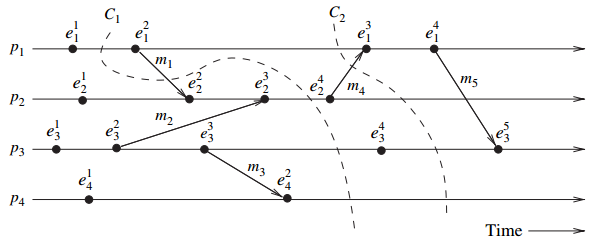一致性分割：在 PAST 接收的消息一定是在 PAST 发送。
图中分割线 $C_1$ 是不一致的；$C_2$ 是一致的。
4.2.4 记录全局快照遇到的问题
(1) 如何判别消息是否会被记录在快照中
快照前的发送消息一定会被记录在全局快照中。如果全局快照包含快照后的发送消息，则不一致。
(2) 如何确定进行快照的瞬间
如果 $p_j$ 接收到的消息 $m_{ij}$ 是在 $p_i$ 进行快照之后发送的，那么 $p_j​$ 先进行快照，然后处理消息。
有两类消息：

计算消息：通过应用程序交换
控制消息：通过快照算法交换，对应用程序透明

4.3 FIFO 通道的快照算法
4.3.1 Chandy - Lamport 算法
$LS_i$ 由 $p_i$ 记录；通道状态 $SC_{ij}$ 由接收方 $p_j​$ 记录。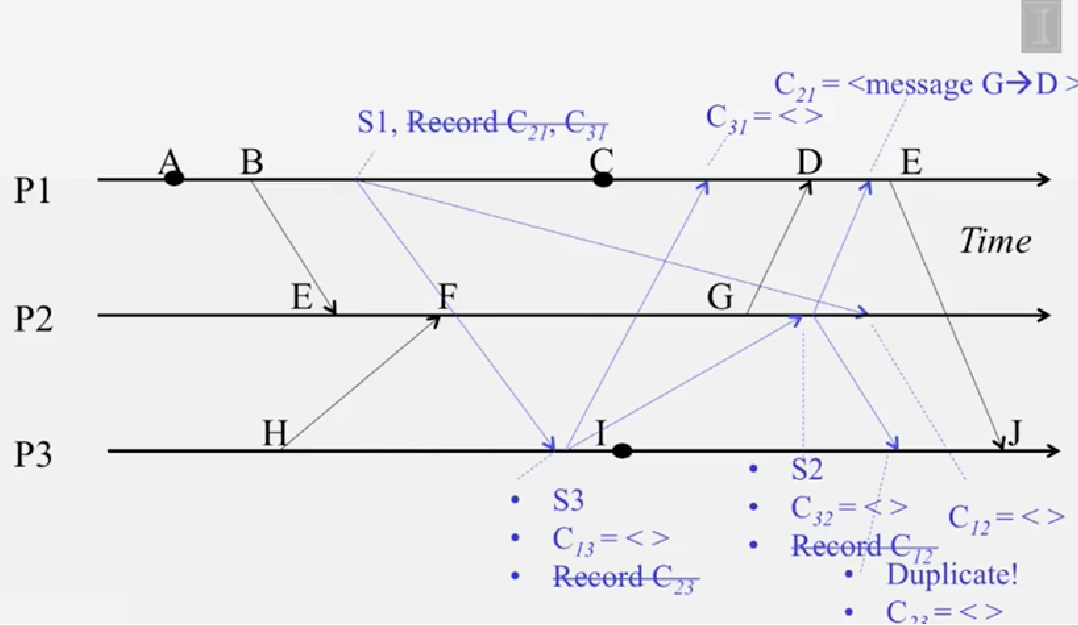进程 $p_i$ 开启快照

记录本地状态 $LS_i​$
开始记录所有通道 $C_{ki}$ 的接收消息（$SC_{ki}$ 初始化为 $\phi$）
向每个输出通道 $C_{ik}​$ 发送标记

进程 $p_i$ 的标记发送规则

记录本地状态 $LS_i​$

向每个输出通道 $C_{ik}​$ 发送标记

进程 $p_j$ 的标记接收规则

$p_j$ 从通道 $C_{ij}$ 接收到标记时：

If $p_j​$ 并未记录本地状态（即第一次接收标记）

记录 $C_{ij}$ 的状态为空集，即 $SC_{ij} = \phi$，这就是 $SC_{ij}$
开始记录通道 $C_{kj},\ k\neq i$ 的接收消息（$SC_{kj}$ 初始化为 $\phi$）
执行“标记发送规则”（与上面操作同步）

Else（即已经接收过标记）

停止记录 $C_{ij}​$ 的消息（当然还是正常处理）
这就是 $SC_{ij}​$

proof:

这翻译真是扯淡。。。

对于“消息守恒”条件：发送事件先于本地标记事件，根据FIFO模型，接收方会在本方发送的标记前收到消息。如果本方标记是接收方的第一个标记，那么接收事件一定属于接收方本地状态；否则本方标记不是接收方的第一个标记，那么消息要么属于通道状态，要么属于接收方本地状态。
对于“因果”条件：发送事件晚于本地标记事件，根据FIFO模型，接收方会在本方发送的标记后收到消息。如果本方标记是接收方的第一个标记，接收方本地快照，快照并未包含接受这个消息的操作，并且通道状态为空，于是消息一定不属于通道状态；否则本方标记不是接收方的第一个标记，通道状态的记录已经停止，不会记录到这个消息。
复杂度：
算法需要 $O(e)$ 个消息和 $O(d)$ 时间，其中 $e=\binom{n}{2}$ 为网络的边，$d​$ 是网络的直径，即最大的两点最短距离。
参考：

Cloud Computing Concepts, Part 1 - 5.2 Global Snapshot Algorithm - Illinois

4.3.2 被记录全局状态的性质
4.4 Chandy - Lamport 算法的变种
4.4.1 Spezialetti - Kearns 算法
将系统划分为若干个组，每个组内自己收集全局状态，组间不传播标记，也不做快照。然后每个组的启动者互相交换快照。（不过我没懂那组间的接收通道的状态怎么来？）
4.4.2 Venkatesan 快照增量算法
适用于需要重复收集系统的全局快照。
每个快照有版本号，修改了标记发送规则。（讲得很粗，不懂）
4.4.3 Helary 波同步方法
4.5 非 FIFO 通道的快照算法
在非FIFO模型中，通道中的消息以随机的方式被接收。需要采取其他技术保证“因果”条件被满足。
4.5.1 Lai - Yang 算法
(1) 每个进程开始是白色的，快照时变为红色。红色进程执行“标记发送规则”
(2) 进程发送的消息的颜色与进程相同。因此，白色消息是快照前发送，红色消息是快照后发送。
(3) 每一个白色进程必须在接收第一个红色消息之前快照
这保证了一个进程在记录了本地快照之后发送的消息（红），不会被未进行快照的接收进程处理。（保证“因果”条件）

FIFO模型自动区分快照前后的消息，但是非FIFO模型不行，必须给消息加入一个tag，用来区分快照前后发送的消息。

(4) 每个白色进程记录了每个通道发送或接收白色消息的历史
(5) 进程变红时，它把历史和自己的快照一起发送给收集全局快照的启动者
(6) 启动者评估 $transit(LS_i, LS_j)$，计算 $C_{ij}$ 的状态：
\begin{aligned} SC_{ij} & = p_i\ 在\ C_{ij}\ 上发送的白消息\ -\ p_j\ 在\ C_{ij}\ 上接收的白消息 \\ & = \{ m_{ij}\ |\ send(m_{ij})\in LS_i \}\ -\ \{ m_{ij}\ |\ rec(m_{ij})\in LS_j \} \\ \end{aligned}
proof:

“消息守恒”条件成立：白色消息要么被接收，要么在通道状态中。

“因果”条件成立：因为红色消息既不在通道状态中，也不在本地状态中。

4.5.2 Li 等人的算法
4.5.3 Mattern 算法
基于向量时钟，通过向量时间戳来判断，在非FIFO模型中，一个发送消息是在快照之前还是之后。（不可比呢？？好像是只比较启动者的那一维时间，所以相当于一个tag标记是否已经快照，换句话说一定可比。。。）
4.6 因果传递系统快照
CO 的性质非常强：$send(m_{ij})\to send(m_{kj})\ \Rightarrow\ rec(m_{ij})\to rec(m_{kj})$
4.6.1 进程状态记录
这两个算法用相同的原理记录本地状态：启动者广播一个标志 $token$（包括自己），进程 $p_i$ 接收到 $token$ 的副本 $token_i$ 时，记录本地快照 $LS_i​$，并发送给启动者。当启动者收到每个进程的快照时，算法终止。
每个进程 $p_i$ 维护：

$SENT_i[1..n]$：$SENT_i[j]$ 是 $p_i$ 发给 $p_j$ 的消息集合
$RECD_i[1..n]$：$RECD_i[j]$ 是 $p_i$ 接收 $p_j$ 的消息数集合

当进程 $p_i$ 收到 $token_i$ 记录本地快照时，将数组 $SENT_i, RECD_i$ 和本地快照一并发给启动者。当算法终止时，启动者按下述方法确定通道状态：
(1) 从启动者到每个进程的通道状态为空
(2) $SC_{ij}$ 是由 $SENT_i[j] - RECD_j[i]$ 给出的消息集合（注意：并不一定有 $RECD_j[i] \subseteq SENT_i[j]​$）
proof:
对于“消息守恒”条件：
对于一个 $send(m_{ij})\in LS_i$

如果 $p_i$ 是启动者，那么一定有 $SC_{ij} = \phi\ \land\ rec(m_{ij})\in LS_j$，因为 $send(m_{ij}) \to send(token_j) \Rightarrow rec(m_{ij}) \to rec(token_j)$
否则要么 $rec(m_{ij})\in LS_j$，要么 $m_{ij}\in SENT_i[j]-RECD_j[i]$

对于“因果”条件：
对于一个 $send(m_{ij})​$ 事件，使得 $send(token) \to rec(token_i) \to send(m_{ij})​$

$send(m_{ij}) \notin SC_{ij}$，因为 $send(m_{ij}) \notin SENT_i[j]$
$rec(token_j) \to rec(m_{ij}) \Rightarrow rec(m_{ij}) \notin LS_j$

这里应该是要假设 “所有 $send(token_x)$ 在同一瞬间完成”

4.6.3 Alagar - Venkatesan 算法中的通道状态记录
定义 旧：$send(m_{ij}) \to send(token_x)$；否则称为新。
按下述方法记录通道状态：
(1) 当进程接收 $token$ 时，进行本地快照，返回 $Done​$ 消息给启动者。并开始记录接收通道上的旧消息
(2) 启动者收到所有 $Done$ 消息后，广播一个终止消息（$send(stop_x)$）
(3) 当收到终止消息，进程停止记录通道状态
proof:
首先注意到一个事实：$send(token_x) \to rec(token_i) \to send(Done_i) \to send(stop_x) \to rec(stop_i)$
对于“消息守恒”条件：
对于一个 $send(m_{ij}) \in LS_i$，有 $send(m_{ij}) \to send(stop_j) \Rightarrow rec(m_{ij}) \to rec(stop_j)$，于是要么 $rec(m_{ij}) \in LS_j$，要么 $m_{ij} \in SC_{ij}$。
对于“因果”条件：
对于一个 $send(m_{ij})$ 事件，使得 $rec(token_i) \to send(m_{ij})$

注意到 $send(m_{ij})$ 是新消息，所以 $m_{ij} \notin SC_{ij}$
$send(token_j) \to send(m_{ij}) \Rightarrow rec(token_j) \to rec(m_{ij})$ 表明 $rec(m_{ij}) \notin LS_j$

快照算法的比较：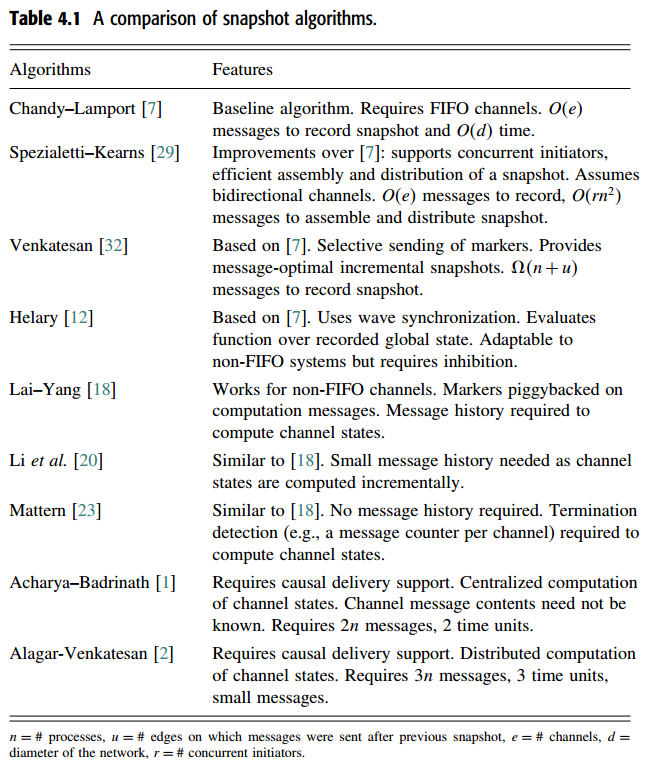4.8 一致性全局快照的必要和充分条件
检查点：进程的中间状态，可以认为没有事件。用 $C_{p,i}$ 表示进程 $p_p$ 的第 $i$ 个检查点。$C_{p,i}$ 所代表的区间由 $C_{p,i-1}$ 到 $C_{p,i}​$ 的所有事件组成。
全局快照就是每个进程上一个检查点的集合。如果全局快照中任何两个检查点之间没有 happens-before relation，则这个全局快照是一致性的。
4.8.1 Zigzag 路径和一致性全局快照
1. Zigzag 路径
定义1：从检查点 $C_{x,i}$ 到 $C_{y,j}$ 存在一条 $zigzag$ 路径，当且仅当存在消息 $m_1,...,m_q​$，使得
(1) $m_1$ 被进程 $p_x$ 在 $C_{x,i}$ 之后发送
(2) 如果 $m_k\ (k\in[1,q-1])$ 被 $p_z$ 接收，那么 $m_{k+1}$ 在相同的（或之后）检查点区间被 $p_z$ 发送。（$send(m_{k+1})$ 和 $rec(m_k)$ 先后关系未指定！！换句话说，如果 $send(m_{k+1})$ 在前，那么一定在同一个检查点区间内；如果 $send(m_{k+1})​$ 在后，则没有要求，自然同步）
(3) $m_q​$ 被进程 $p_y​$ 在 $C_{y,j}​$ 之前接收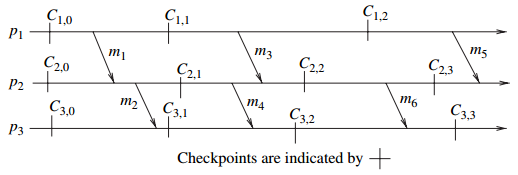例如：$C_{1,1}$ 到 $C_{3,2}$ 的 $zigzag$ 路径经过 $m_3$ 和 $m_4$。（进程 $p_2$ 在同一检查点区间内，$rec(m_3)$ 并且 $send(m_4)$，另外 $send(m_4)$ 先于 $rec(m_3)$）
定义2：一个检查点 $C$ 被包含在一个 $zigzag$ 环路中，当且仅当存在一条从 $C$ 到本身的 $zigzag​$ 路径。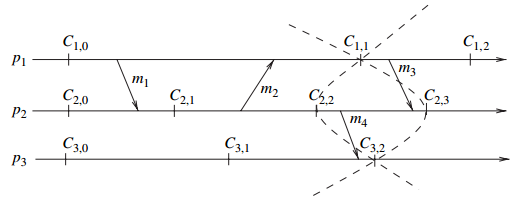例如：$C_{2,1}$ 是在由消息 $m_1$ 和 $m_2$ 形成的一条 $zigzag$ 环路中。（消息序列为 $m_2, m_1$；在进程 $p_2$ 中，$m_2$ 在检查点 $C_{2,1}$ 之后发送；在进程 $p_1$ 中，同一检查点内 $send(m_1)$ 先于 $rec(m_2)$；最后在进程 $p_2$ 中，$m_1$ 在 $C_{2,1}$ 之前被接收）
2. Zigzag 路径和因果路径之间的不同
因果路径：存在一条从检查点 $A$ 到检查点 $B$ 的因果路径，当且仅当存在一条在 $A$ 之后开始，在 $B$ 之前结束的消息链，该链中前一个消息被接收后，后一个消息才被发送。因果路径是 $zigzag$ 路径。
在 $zigzag$ 路径中，消息链的前一个消息接收之前，后一个消息允许已经被发送，只要在同一个检查点区间内。
3. 一致性全局快照
$zigzag$ 路径的性质：任何包含这两个检查点的快照都是不一致性的。
Netzer 和 Xu 证明：如果检查点集合 $S$ 是一个一致性全局快照，当且仅当 $S$ 中任意两点不存在 $zigzag​$ 路径。总结：

在快照的检查点之间没有因果路径是一致性快照的必要条件
在快照的检查点之间没有 $zigzag$ 路径是一致性快照的充分必要条件
一个检查点能够成为一致性快照的一部分，当且仅当该检查点不属于 $zigzag$ 环路

4.9 找出分布式计算中的一致性全局快照
定义：$A​$ 和 $B​$ 是检查点，$R​$ 和 $S​$ 是检查点集合，令 $\leadsto​$ 是检查点和检查点集合间的关系
(1) $A\leadsto B$，当且仅当存在从 $A$ 到 $B$ 的 Z 路径
(2) $A\leadsto S$，当且仅当存在从 $A$ 到 $S$ 的某个成员的 Z 路径
(3) $S\leadsto A$，当且仅当存在一条从 $S$ 的某个成员到 $A$ 的 Z 路径
(4) $R\leadsto S​$，当且仅当存在一条从 $R​$ 的某个成员到 $S​$ 的某个成员的 Z 路径
定理：检查点集合 $S$ 能被扩展成一个一致性全局快照，当且仅当 $S\not\leadsto S$
4.9.1 找出一致性全局快照
令 $S$ 是使得 $S\not\leadsto S$ 的检查点的一个集合，那么对进程 $p_q$，集合 $S_{useful}^q$ 被定义为
$S_{useful}^q = \{ C_{q,i}\ |\ (S\not\leadsto C_{q,i})\ \land\ (C_{q,i}\not\leadsto S)\ \land\ (C_{q,i}\not\leadsto C_{q,i}) \}$
之后定义 $S_{useful} = \bigcup_q S_{useful}^q$
引理：令 $S$ 是使得 $S\not\leadsto S$ 的检查点的一个集合，并且 $C_{q,i}\notin S$。那么 $S\cup \{C_{q_i}\}$ 能扩展成一个一致性快照，当且仅当 $C_{q,i}\in S_{useful}$。
定理：令 $S​$ 是使得 $S\not\leadsto S​$ 的检查点的一个集合，且 $T​$ 是任意检查点集合满足 $T \cap S = \phi​$。那么 $S\cup T​$ 是一个一致性全局快照，当且仅当

$T\subseteq S_{useful}$
$T\not\leadsto T$
$|S\cup T| = N​$

4.9.2 枚举式一致性快照 Manivannan - Netzer - Singhal 算法
\begin{aligned} & ComputeAllCgs(S)\ \{ \\ & \qquad \textbf{let}\ G = \phi \\ & \qquad \textbf{if}\ S\not\leadsto S\ \textbf{then} \\ & \qquad \qquad 设\ AllPros\ 表示未在集合\ S\ 中出现的进程 \\ & \qquad \qquad ComputeAllCgsFrom(S,\ AllPros) \\ & \qquad \textbf{return}\ G \\ & \} \\ & \\ & ComputeAllCgsFrom(S, ProcSet)\ \{ \\ & \qquad \textbf{if}\ (ProcSet = \phi)\ \textbf{then} \\ & \qquad \qquad G = G\cup \{S\} \\ & \qquad \textbf{else}\ \\ & \qquad \qquad 设\ P_q\ 表示集合\ ProcSet\ 中的任一进程 \\ & \qquad \qquad \textbf{for}\ \forall\ C\in S_{useful}^q\ \textbf{do}\ \\ & \qquad \qquad \qquad ComputeAllCgsFrom(S\cup\{C\},\ ProcSet - \{P_q\}) \\ & \} \\ \end{aligned}
$ComputeAllCgs(S)$ 返回包含 $S$ 的所有一致性快照的集合。（即 $G$ 中的每一个元素都是包含 $S$ 的一致性快照）
$ComputeAllCgsFrom(S, ProcSet)$ 通过递归，试图从每一个可扩展的检查点向下遍历。
proof：略。
4.9.3 在分布式计算中找出 Z 路径
定义：分布式计算的回滚依赖图（R图）是一个有向图 $G=(V,E)$，其中 $V$ 是检查点集合，边 $(C_{p,i}, C_{q,j})\in E$ 如果
(1) $p=q\ \land\ j=i+1$ 或
(2) $p\neq q$ 且 $C_{p,i}$ 区间发送的消息被 $C_{q,j}​$ 区间接收
构建一个R图：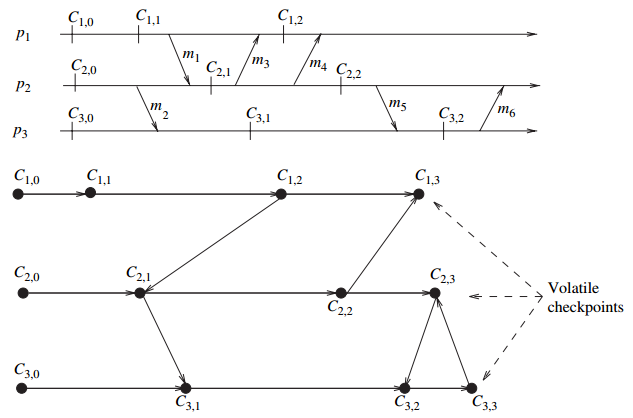定理：令 $G=(V,E)$ 是R图，那么对任意两个检查点 $C_{p,i}$ 和 $C_{q,j}$，有 $C_{p,i} \overset{\text{rd}}{\leadsto} C_{q,j}$ 当且仅当
(1) $p=q\ \land\ i,j​$ 或
(2) $C_{p,i+1}\overset{\text{rd}}{\leadsto} C_{q,j}$（可能有 $p=q$）
4.10 本章小结
记录全局快照的一个重点在于区分本地快照前后发送的消息，在不同通信模型下会有不一样的处理。


展开全文• ## 什么是分布式计算

千次阅读 2012-04-21 21:04:53
分布式计算是一门计算机科学，主要研究分布式系统。一个分布式系统包括若干通过网络互联的计算机。这些计算机互相配合以完成一个共同的目标（我们将这个共同的目标称为“项目”）。具体的过程是：将需要进行大量计算...
分布式计算是一门计算机科学，主要研究分布式系统。一个分布式系统包括若干通过网络互联的计算机。这些计算机互相配合以完成一个共同的目标（我们将这个共同的目标称为“项目”）。具体的过程是：将需要进行大量计算的项目数据分割成小块，由多台计算机分别计算，再上传运算结果后统一合并得出数据结论。在分布式系统上运行的计算机程序称为分布式计算程序；分布式编程就是编写上述程序的过程。

中国科学技术信息研究所的定义
分布式计算是近年提出的一种新的计算方式。所谓分布式计算就是在两个或多个软件互相共享信息，这些软件既可以在同一台计算机上运行，也可以在通过网络连接起来的多台计算机上运行。分布式计算比起其它算法具有以下几个优点：

1、稀有资源可以共享。

2、通过分布式计算可以在多台计算机上平衡计算负载。

3、可以把程序放在最适合运行它的计算机上。

其中，共享稀有资源和平衡负载是计算机分布式计算的核心思想之一。
网格计算
实际上，网格计算就是分布式计算的一种。如果我们说某项工作是分布式的，那么，参与这项工作的一定不只是一台计算机，而是一个计算机网络，显然这种“蚂蚁搬山”的方式将具有很强的数据处理能力。网格计算的实质就是组合与共享资源并确保系统安全。

百度知道：http://baike.baidu.com/view/30655.htm

展开全文分布式计算 网络 算法
• 在刚开始选题时，我并没有想写分布式计算框架，只是想试试N皇后算法如何分布式计算，结果随着版本的迭代变成了分布式计算框架。一、综述 随着大数据时代的来临，现有计算方式已不能满足工作需求，并且CPU近几年往...
    大学时光飞逝，转眼就到大四。毕业设计作为在大学编写的最后一个程序必须精益求精。在刚开始选题时，我并没有想写分布式计算框架，只是想试试N皇后算法如何分布式计算，结果随着版本的迭代变成了分布式计算框架。一、综述    随着大数据时代的来临，现有计算方式已不能满足工作需求，并且CPU近几年往多核方面发展，单台电脑性能有限不足以完成复杂计算任务。分布式计算框架能很好的解决此类需要巨大计算量问题，分布式计算框架允许使用商用服务器组成一个计算集群并提供一个并行计算软件框架，服务器之间的通信、负载均衡、任务计算与处理、任务储存等复杂的操作都交由系统自动处理，减少了软件开发人员的负担。    本系统是一个基本C++平台的分布式计算框架，使用了Digia公司开发工具QT5.10；任务储存使用了开源数据库MYSQL。该分布式框架实现了动态链接库的上传、任务文件上传、任务文件解析、计算任务的分发、计算任务的计算、计算结果的储存、计算结果的汇总等功能。在分配任务过程中，本框架优先分配给最先执行完任务的计算节点，高效利用计算资源。当计算节点断开连接时，本框架会自动将其任务重置，并分发给其他计算节点。使用了任务队列与任务报错等容错手段保证了分布式计算框架的稳定运行。    用户编写模板对应函数Read、Handle、Sum，生成动态链接库后上传至服务器中，随后将任务文件上传即可完成整个分布式计算过程。服务器调用Read函数对任务文件进行任务读取与分解，随后将分解任务分配至空闲计算节点，计算节点调用Handle函数进行任务计算，计算完毕后回传任务结果至服务器，当整个计算任务完成时服务器调用Sum函数进行任务的汇总，最后完成分布式任务的计算。(一）、系统结构    系统结构大致如下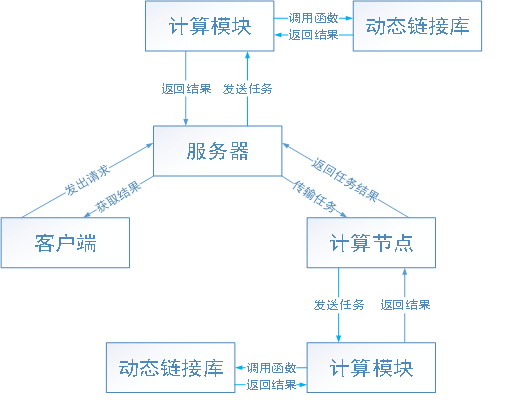1、服务器模块     服务器模块负责接收转发来自客户端、计算模块、计算节点发送的数据并调用相关函数进行处理。当服务器接收动态链接库时会保存并转发给所有计算节点。接收计算任务时则调用计算模块中的Read函数，获取分解任务后开始分发至计算节点开始计算。当所有计算任务完成时，服务器调用计算模块中的Sum函数，获取汇总结果后保存至数据库。2、计算节点模块     计算节点负责监控其机器实时状态，并作为守护程序监控计算模块程序实时状态，在其崩溃时做出相应响应，并且负责传递计算任务给计算模块。3、客户端模块    客户端模块是一个与系统交互的模块，当用户查询相关数据时，客户端将请求数据发送给服务器，服务器处理任务将数据发送给客户端，客户端接收并显示数据。用户编写好动态链接文件与任务文件，客户端将其传输至服务器即可开始分布式计算。4、计算模块    计算模块负责处理计算任务，调用相应动态链接库的相应函数进行任务的计算，计算完毕后传输数据给计算节点。为了防止因动态链接库原因导致所有程序崩溃，本系统将计算模块划分出一个单独的程序。即使因动态链接库原因使计算模块崩溃，系统也可重新启动计算模块并上报至服务器，提高了系统的稳定性。（二）、设计性能       性能测试有误差，仅供参考（云服务器太贵测试不起）     （一）压力测试    在配置为2核(intel E5  2.29GHZ) 、内存2G、网络带宽1M、系统Windows Server2012的云服务器上进行性能测试中，程序平均每秒分发处理600个任务，CPU平均利用率50%，内存占用随着任务数的减小而下降，IO读写频繁。（带宽为瓶颈）      （二）与Hadoop性能对比测试    本次性能测试算法采用N皇后算法，在四台虚拟机中进行性能测试。单个虚拟机配置为单核 内存768M 网络带宽100M 。Hadoop测试采用CetOS7.3系统，本程序采用Win7。测试算法内容为18阶皇后，在不进行分布式算法处理计算耗时为986秒。为了形成对比，将18阶皇后分解任务数分为：321700、36264、3420、272。测试结果如下（这个结果我测了几次每次都不一样，太费时间就这样吧）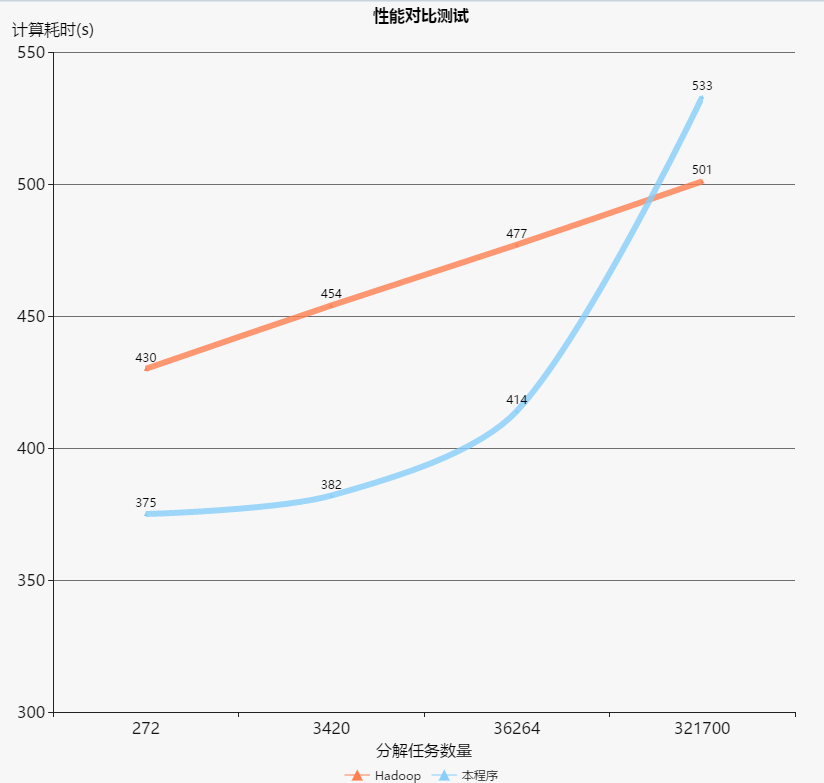（三）效率测试    采用19阶皇后算法进行测试，将相同计算量的任务分配给不同数量的计算节点，通过计算时间分析整个分布式计算框架的计算效率。为了更好做出对比，以节点个数为1的计算时间为基准计算时间，那么理想计算消耗时间为：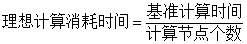计算效率为：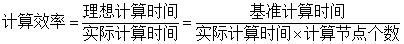以下是计算效率折线图（看看就行）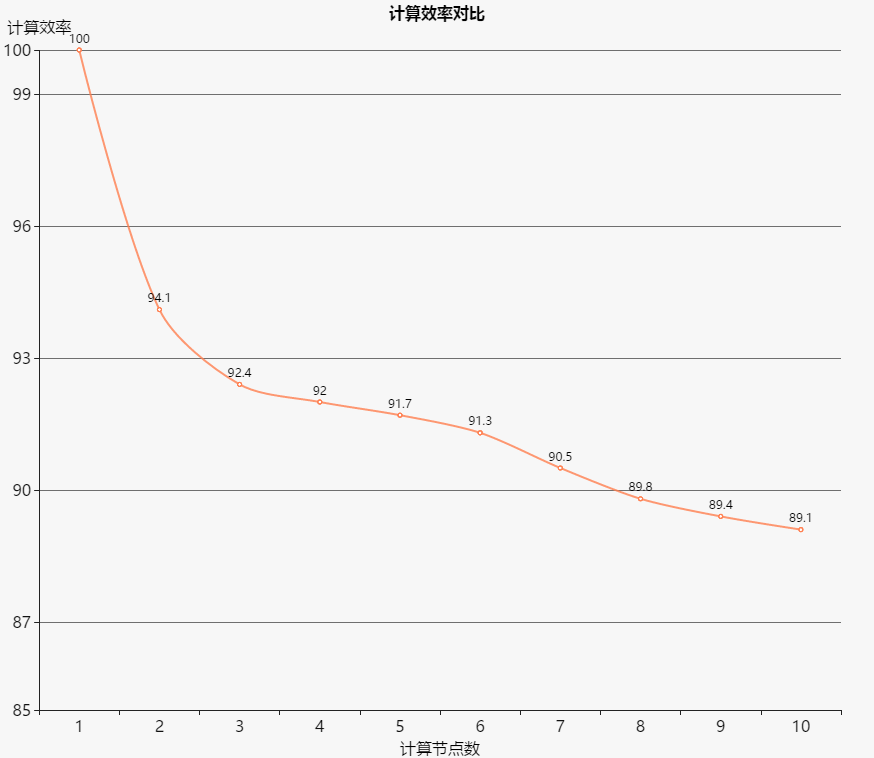（三）、主要界面源码地址：点击打开链接
展开全文• 分布式计算的基本原理
                分布式计算的基本原理转载时请注明出处：http://blog.csdn.net/absurd 从最近几次MMI设计会议讨论的结果来看，嵌入式程序员对于分布式计算知之甚少。他们对分布式计算有种恐惧，所以对分布式架构极力排斥。而他们的人数又占绝对优势，讨论N次，MMI的架构还是没有确定下来。分布式计算已经进入桌面环境，不是企业应用的专利了，像GNOME(GNU Network Object Model Environment)的名字本身就暗示着分布式计算了。本文介绍一下分布式的基本原理，揭开分布式神秘的面纱，让嵌入式程序员熟悉一下。 分布式计算可以分为以下几类： 传统的C/S模型。如HTTP/FTP/SMTP/POP/DBMS等服务器。客户端向服务器发送请求，服务器处理请求，并把结果返回给客户端。客户端处于主动，服务器处于被动。这种调用是显式的，远程调用就是远程调用，本地调用就是本地调用，每个细节你都要清楚，一点都含糊不得。 集群技术。近年来PC机的计算能力飞速发展，而服务器的计算能力，远远跟不上客户端的要求。这种多对一的关系本来就不公平，人们已经认识到靠提高单台服务器的计算能力，永远满足性能上的要求。一种称集群的技术出现了，它把多台服务器连接起来，当成一台服务器来用。这种技术的好处就是，不但对客户来说是透明的，对服务器软件来说也是透明的，软件不用做任何修改就可以在集群上运行。集群技术的应用范围也仅限于此，只能提高同一个软件的计算能力，而对于多个不同的软件协同工作无能为力。 通用型分布式计算环境。如CORBA/DCOM/ RMI/ DBUS等，这些技术(规范)差不多都有具有网络透明性，被调用的方法可能在另外一个进程中，也可能在另外一台机器上。调用者基本上不用关心是本地调用还是远程调用。当然正是这种透明性，造成了分布式计算的滥用，分布式计算用起来方便，大家以为它免费的。实际上，分布式计算的代价是可观的，据说跨进程的调用，速度可能会降低一个数量级，跨机器的调用，速度可能降低两个数量级。一些专家都建议减少使用分布式计算，即使要使用，也要使用粗粒度的调用，以减少调用的次数。 还其一些混合形式(SOAP?)，这里不再多说。我们主要介绍第三种分布式模型，这类分布式模型即适用于企业级应用，也适用于桌面应用。有的专注于企业级应用（如CORBA），有的专注于桌面环境（如DBUS）。它们的实现原理都差不多，基本上都基于传统的RPC或者仿RPC实现的，下面介绍一下它们的基本原理。 我们先看一下分布式的最简模型：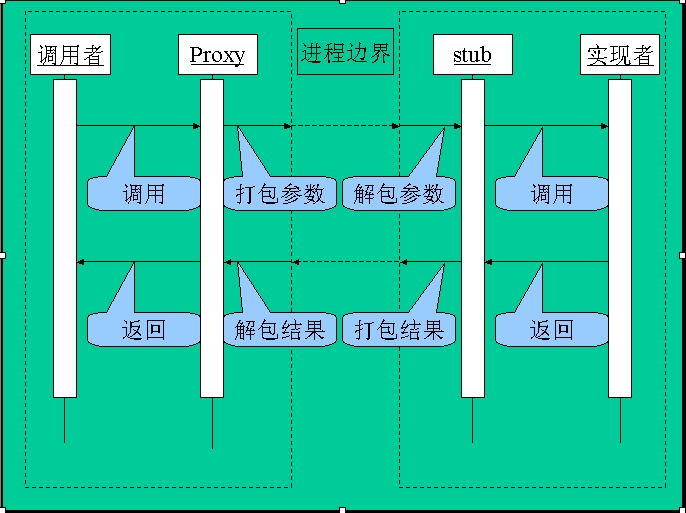在传统的方法中，调用一个对象的函数很简单：创建这个对象，然后调用它的函数就行了。而在分布式的环境中，对象在另外一个进程中，完全在不同的地址空间里，要调用它的函数可能有点困难了。 看看传统的C/S模型的请求方式，客户端把参数通过网络发给服务器，服务器根据参数要求完成相应的服务，然后把结果返回给客户端，客户端拿到结果了，一次请求算完成。由此看来，调用远程对象似乎并不难，问题在于这种方式不是网络透明的，每一个细节你都要自己处理，非常复杂。 要简化软件的设计，当然是网络操作透明化，调用者和实现者都无需关心网络操作。要做到这一点，我们可以按下列方法： 在客户端要引入一个代理(Proxy)对象。它全权代理实际对象，调用者甚至都不知道它是一个代理，可以像调用本地对象一样调用这个对象。当调用者调用Proxy的函数时，Proxy并不做实际的操作，而是把这些参数打包成一个网络数据包，并把这个数据包通过网络发送给服务器。 在服务器引入一个桩(Stub)对象，Stub收到Proxy发送的数据包之后，把数据包解开，重新组织为参数列表，并用这些参数就调用实际对象的函数。实际对象执行相关操作，把结果返回给Stub，Stub再把结果打包成一个网络数据包，并把这个数据包通过网络发送给客户端的Proxy。 Proxy收到结果数据包后，把数据包解开为返回值，返回给调用者。至此，整个操作完成了。怎么样，简化吧。 Proxy隐藏了客户端的网络操作，Stub隐藏了服务器端的网络操作，这就实现了网络透明化。你也许会说，根本没有简化，只是把网络操作隔离开了，仍然要去实现Proxy和Stub两个对象，一样的麻烦。 没错。不过仔细研究一下Proxy和Stub的功能，我们会发现，对于不同对象，这些操作都差不多，无非就是打包和解包而已，单调重复。单调重复的东西必然有规律可循，有规律可循就可以用代码产生器自动产生代码。 像DCOM和CORBA等也确实是这样做的，先用IDL语言描述出对象的接口，然后用IDL编译器自动产生Proxy和Stub代码，整个过程完全不需要开发人员操心。 打包和解包的专业术语叫做marshal和unmarshal，中文常用翻译为列集和散集。不过这两个词太专业了，翻译成中文之后更加让人不知所云。我想还是用打包和解包两个词更通俗一点。 在以上模型中，调用对象的方法，确实做到了网络透明化。读者可以会问，我要访问对象的属性怎么办呢？对象的属性就是变量，变量就一块内存区域，内存区域在不同的进程里完全是独立的，这看起来确实是一个问题。还记得很多关于软件设计书籍里面讲过的吗：不要暴露对象属性，调用者若要访问对象的属性，通过get/set方法去访问。这样不行了吗，对属性的访问转换为对对象方法的调用。 OK，调用对象的方法和访问对象的属性都解决了。还有重要的一点，如何创建对象呢。因为实际的对象并不固定在某台机器上，它的位置可能是动态的。甚至Proxy本身也不知道Stub运行在哪里。如果要让调用者来指定，创建对象的过程仍未达到网络透明化。通常的做法是引入一个第三方中介，这个第三方中介是固定的，可以通过一定的方法找到它。第三方中介负责在客户端的Proxy和服务器的Stub之间穿针引线。第三方中介通常有两种：一种是只负责帮客户端找到服务器，之后客户端与服务器直接通信。另外一种就是不但负责找到服务器，而且负责转发所有的请求。 以上的模型仍然不完整，因为现实中的对象并不是一直处理于被动的地位。而是在一定的条件下，会主动触发一些事件，并把这些事件上报给调用者。也就是说这是一个双向的动作，单纯的C/S模型无法满足要求，而要采用P2P的方式。原先的客户端同时作为一个服务器存，接受来自己服务器的请求。像COM里就是这样做的，客户端要注册对象的事件，就要实现一个IDispatch接口，给对象反过来调用。 自己实现时还要考虑以下几点： l         传输抽象层。分布可能是跨进程也可能是跨机器。在不同的情况下，采用不同的通信方式，性能会有所不同。做一个传输抽象层，在不同的情况下，可选用不同的传输方式，是一种好的设计。 l         文本还是二进制。把数据打包成文本还是二进制？打包成文本的好处是，可移植性好，由于人也可以看懂，调试方便。坏处是速度稍慢，打包后的数据大小会明显变大。采用二进制的好处是，速度快，打包后的数据大小与打包前相差不大。坏处是不易调试，可移植性较差。 l         字节顺序和字节对齐。若采用二进制方式传输，可移植性是个问题。因为不同的机器上，字节顺序和字节对齐的方式都有些差异，在数据包中要加入这些说明，以提高可移植性。               再分享一下我老师大神的人工智能教程吧。零基础！通俗易懂！风趣幽默！还带黄段子！希望你也加入到我们人工智能的队伍中来！https://blog.csdn.net/jiangjunshow


展开全文• 整理自《架构解密从分布式到微服务》第七章——聊聊分布式计算。 前言 不管是网络、内存、还是存储的分布式，它们最终目的都是为了实现计算的分布式：数据在各个计算机节点上流动，同时各个计算机节点都能以某种...分布式计算 MapReduce Storm Spark
• **分布式计算是当前计算机领域常见的名词，那么到底什么是分布式，什么又是分布式计算呢？今天和大家共同研究一下这个话题。** 分布式计算的概念 一个分布式系统是由若干通过网络互联的计算机组成的软硬件系统，且...
• 分布式计算 目录[隐藏] 什么是分布式计算分布式计算的意义和格局 BOINC分布式计算平台介绍 分布式计算安全吗？ 分布式计算在中国 什么是分布式计算？　所谓分布式计算是一门计算机科学，它研究如何把一个需要非常...
• ## 分布式计算概念

千次阅读 2018-06-09 16:12:18
1.分布式计算的定义 分布式计算是一门计算机科学，主要研究对象是分布式系统。 分布式系统是由若干通过网络互联的计算机组成的软硬件系统，且这些计算机互相配合以完成一个共同的目标（往往这个共同的目标...
• 3、黄金搭档：分布式存储+分布式计算 这篇文章聊一个话题：什么是分布式计算系统？ （1）从一个新闻门户网站案例引入 现在很多同学经常会看到一些名词，比如分布式服务框架，分布式系统，分布式存储系统...分布式存储 分布式计算 Spark
• 分布式计算的概述什么是分布式计算分布式计算的有点及缺点优点缺点分布式计算的相关计算形式分布式系统概述分布式系统的特征分布式计算的基础技术 什么是分布式计算 什么是分布式计算呢，顾名思义分布式计算就是多...云计算 大数据 分布式计算 分布式系统 进程间通信
• 分布式计算是一种计算方法，和集中式计算是相对的。随着计算技术的发展，有些应用需要非常巨大的计算能力才能完成，如果采用集中式计算，需要耗费相当长的时间来完成。分布式计算将该应用分解成许多小的部分，分配给...阿里云大学 大数据 分布式计算
• 分布式计算框架与分布式文件系统是两个概念。分布式计算框架是用于处理大数据的一种模型，而分布式文件系统可以用于大数据的存储。 一、分布式计算框架 对于如何处理大数据，计算机科学界有两大方向：一是集中式计算...
• 分布式计算 分布式计算是利用互联网上的计算机的中央处理器的闲置处理能力来解决大型计算问题的一种计算科学。研究如何把巨大的问题分成许多小的部分，然后把这些小任务分配给许多计算机进行处理，最后把这些计算...云计算 分布式计算 网格计算
• 分布式计算、云计算与大数据 第一章分布式计算的概念定义分布式计算的优缺点分布式计算的相关计算形式分布式系统概述分布式系统的定义分布式系统的特征分布式计算的基础技术进程间通信IPC程序接口原型时间同步死锁和...
• 周末抽空看了看分布式计算和并行计算方面的东西，主要是搞清楚了这两个东西的相似点和区别，随便记录几句。相似点很简单，都是为了实现比较复杂的任务，将大的任务分解成小的任务，在多台计算机上同时计算。麻烦的是...分布式计算 并行计算
• 什么是分布式计算 基本概念 和集中式计算相反，分布式计算的一个计算过程将会在多台机器上进行。组件之间彼此进行交互以实现一个共同的目标，把需要进行大量计算的工程数据分区成小块，由多台计算机分别计算，再...Spark 分布式计算
• 主要内容来自维基百科 先上一张图大略直观感受一下： 分布式系统是联网计算机组，其...并行计算可以被看作分布式计算的一个特定的紧密耦合的形式，和分布式计算可以被视为并行计算的松散耦合形式。...
• 云计算与分布式计算区别 分布式计算是指在一个松散或严格约束条件下使用一个硬件和软件系统处理任务，这个系统包含多个处理器单元或存储单元、多个并发的过程、多个程序。一个程序被分成多个部分，同时在通过网络...
• 分布式计算，网格计算和云计算的异同 分布式计算：研究如何把一个需要非常巨大的计算能力才能解决的问题分成许多小的部分，然后把这些部分分配给许多计算机进行处理，最后把这些计算结果综合起来得到最终的结果。 ...分布式计算 网格计算 云计算 网格
• 之前就在想，分布式计算既然这么厉害，为什么还会需要超算呢，就从网上看了一些资料。 分布式计算工作原理 分布式计算是利用互联网上的计算机的中央处理器的闲置处理能力来解决大型计算问题的一种计算科学。...技术
...